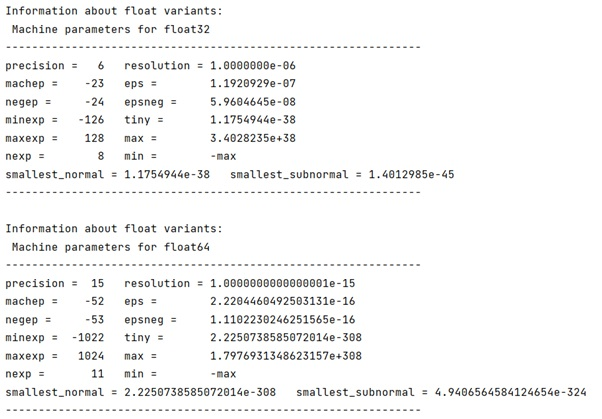# How to check the size of a float?

Learn, how can we swap two rows of an array using NumPy?
Submitted by Pranit Sharma, on March 27, 2023

## Overview

Basically, data types are the category of values or types of values that can be used in programming for computations. To store different types of values (integer, decimal, characters, etc.), we need different data types in Python or any other programming language.

## Float Type

The Float is a data type that is used to store decimal values, however, the catch is float itself is categorized into different forms and all of them are having their own sizes.

For example, we have float32, float64, etc that have a different range to store the decimal values. All the properties of a Python float can be requested via sys.float_info(). It returns information such as max/min value, max/min exp value, etc.

## Checking the size of float

However, a much easier approach to find the information about the float size is to use numpy.finfo() which is powerful enough to tell us about the properties of float or any other data type including the number of bits in the exponents, the number of bits in the mantissa, etc.

Let us understand with the help of an example,

## Python code to check the size of a float

```# Import numpy
import numpy as np

for f in (np.float32, np.float64):
finfo = np.finfo(f)
# Display result
```

### Output